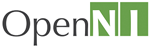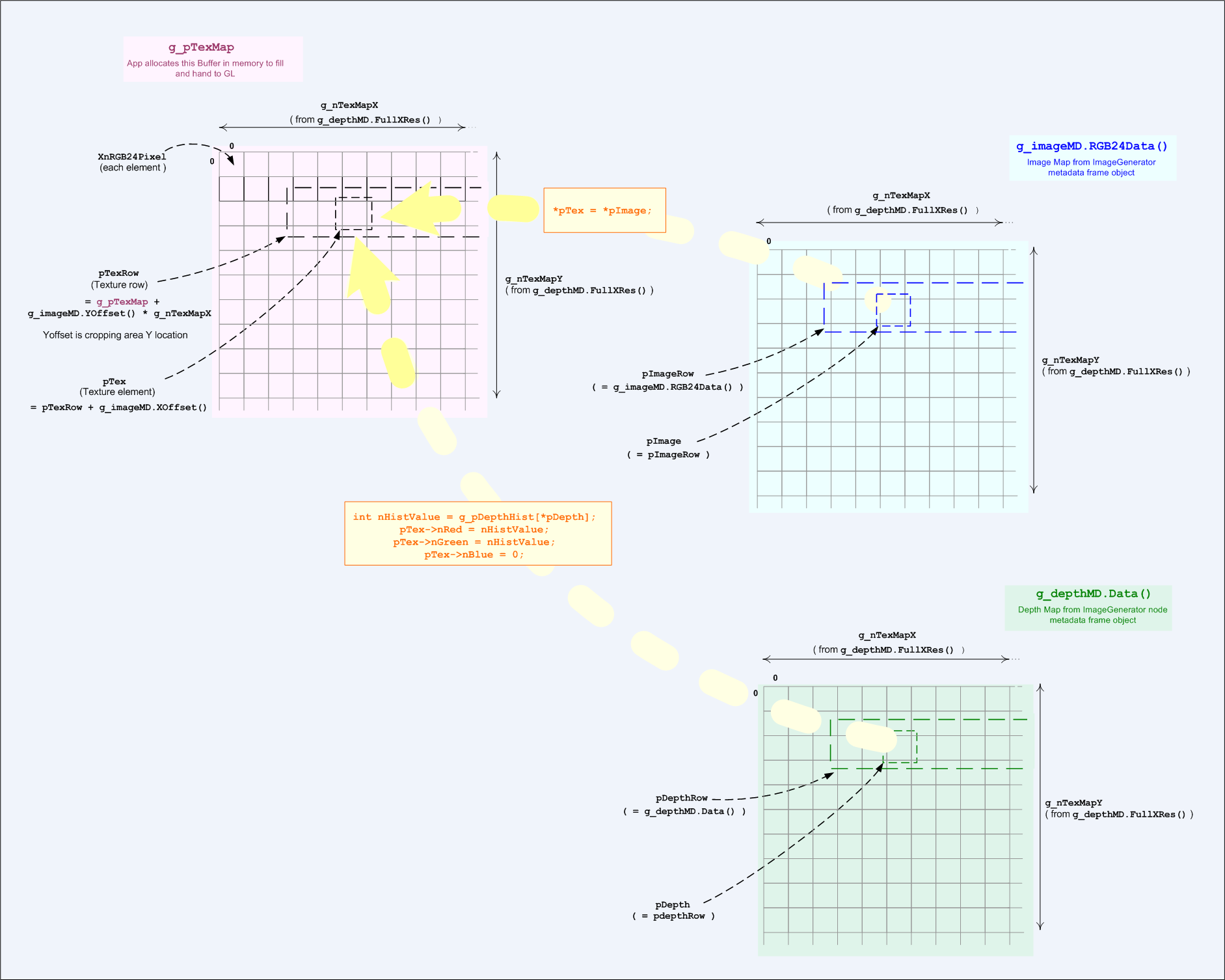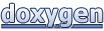OpenNI 1.5.4: NiSimpleViewer.cpp - sample program

OpenNIOpenNI 1.5.4
NiSimpleViewer.cpp - sample program

Source file: Click the following link to view the source code file:

• NiSimpleViewer.cpp

This section describes the SimpleViewer sample program. This sample program uses a DepthGenerator node and ImageGenerator node to build an accumulative histogram from depth values.

Global Declaration Block

The following definition is for the path to an OpenNI XML script file for inputting and building a stored production graph. The production graph is a network of production nodes and is the principal OpenNI object model. The identifies blobs as hands or human users.

#define SAMPLE_XML_PATH "../../../../Data/SamplesConfig.xml"

The following declares the array for the histogram array that is a key part of this sample program. (This is not OpenNI specific.)

float g_pDepthHist[MAX_DEPTH];

The following declaration block declares the OpenNI objects required for building the OpenNI production graph.

Context g_context;
ScriptNode g_scriptNode;
DepthGenerator g_depth;
ImageGenerator g_image;

Each of these declarations is described separately in the following paragraphs.

A Context object is a workspace in which the application builds an OpenNI production graph.

The ScriptNode object loads an XML script from a file or string, and then runs the XML script to build a production graph.

The DepthGenerator node generates a depth map. Each map pixel value represents a distance from the sensor.

The ImageGenerator node generates color image maps of various formats, such as the RGB24 image format. Call its SetPixelFormat() method to set the image format to be generated.

The DepthMetaData object provides a frame object for the xn::DepthGenerator node. A generator node's frame object contains a generated data frame and all its associated properties. This frame object, comprising the data frame and its properties, is accessible through the node's metadata object.

The ImageMetaData object provides a frame object for the xn::ImageGenerator node. This metadata object is associated with an ImageGenerator node in the same way as the DepthMetaData object is associated with a DepthGenerator node.

Main Program

The declarations at the top of the main program collect and report status and errors from any of the OpenNI functions.

XnStatus rc;
EnumerationErrors errors;

Use Script to Set up a Context and Production Graph

The InitFromXmlFile() method is a shorthand combination of two other initialization methods — Init() and then RunXmlScriptFromFile() — which initializes the context object and then creates a production graph from an XML file. The XML script file describes all the nodes you want to create. For each node description in the XML file, this method creates a node in the production graph.

rc = g_context.InitFromXmlFile(SAMPLE_XML_PATH, g_scriptNode, &errors);

Verify Existence of Nodes in the Sample Script File

This is verification code to check that OpenNI found at least one node definition in the script file. The program continues execution only if at least one node definition if found.

if (rc == XN_STATUS_NO_NODE_PRESENT)
{
XnChar strError;
errors.ToString(strError, 1024);
printf("%s\n", strError);
return (rc);
}
else if (rc != XN_STATUS_OK)
{
printf("Open failed: %s\n", xnGetStatusString(rc));
return (rc);
}

Get a DepthGenerator Node from the Production Graph

Assuming that the above call to InitFromXmlFile() succeeded, a production graph is then created.

The FindExistingNode() method in the following code block tries to get a reference to any one of the production nodes. This call specifies XN_NODE_TYPE_DEPTH to get a reference to a DepthGenerator node. A DepthGenerator node generates a depth map as an array of pixels, where each pixel is a depth value representing a distance from the sensor in millimeters. A reference to the node is returned in the depth parameter.

rc = g_context.FindExistingNode(XN_NODE_TYPE_DEPTH, g_depth);

The code block that follows the FindExistingNode() call just checks that OpenNI found a DepthGenerator node in the production graph.

if (rc != XN_STATUS_OK)
{
printf("No depth node exists! Check your XML.");
return 1;
}

Get a DepthGenerator Node from the Production Graph

The following code is similar to the previous code block, but this time the FindExistingNode() method call gets a reference to an ImageGenerator node. Assuming that an ImageGenerator node was found, a reference to it is returned in the g_image parameter.

rc = g_context.FindExistingNode(XN_NODE_TYPE_IMAGE, g_image);
if (rc != XN_STATUS_OK)
{
printf("No image node exists! Check your XML.");
return 1;
}

Get the DepthGenerator's Data

The following statement gets the latest generated depth frame object, saving it as a metadata object.

Get the ImageGenerator's Latest Data

This works the asme as for the DepthGenerator as above.

Checking for Unsupported Mode or Format

The following code block checks for Hybrid mode requirement. Hybrid mode isn't supported in this sample. This check accesses some attributes of the frame data's associated configuration properties: FullXRes() and FullYRes() are the full frame resolution, i.e., the entire field-of-view, ignoring cropping of the FOV in the scene.

if (g_imageMD.FullXRes() != g_depthMD.FullXRes() || g_imageMD.FullYRes() != g_depthMD.FullYRes())
{
printf ("The device depth and image resolution must be equal!\n");
return 1;
}

The following code block checks that the selected pixel format is RGB24. Other formats are not supported. if (g_imageMD.PixelFormat() != XN_PIXEL_FORMAT_RGB24)

if (g_imageMD.PixelFormat() != XN_PIXEL_FORMAT_RGB24)
{
printf("The device image format must be RGB24\n");
return 1;
}

Initializing the Texture Map

The dimensions of the Texture Map buffer are calculated by rounding the full frame resolution of the DepthGenerator data frame. full frame resolution is accessed through xn::MapMetaData::FullXRes() "FullXRes()" and xn::MapMetaData::FullYRes() "FullYRes()" (again, both accessed through the metadata frame object).

g_nTexMapX = (((unsigned short)(g_depthMD.FullXRes()-1) / 512) + 1) * 512;
g_nTexMapY = (((unsigned short)(g_depthMD.FullYRes()-1) / 512) + 1) * 512;
g_pTexMap = (XnRGB24Pixel*)malloc(g_nTexMapX * g_nTexMapY * sizeof(XnRGB24Pixel));

glutDisplay() - Display Control

Significant OpenNI programming is performed inside the glutDisplay() callback.

The following code blocks read the frame objects from the DepthGenerator and ImageGenerator nodes.

the WaitAnyUpdateAll() method in the following statement updates all generator nodes in the context that have new data available, first waiting for a specified node to have new data available. The application can then get the data (for example, using a metadata GetData() method). This method has a timeout.

nRetVal = context.WaitOneUpdateAll(depth);

The following code block calls the GetMetaData() methods of each of the two generator nodes to get the nodes' frame data from the frame objects – depthMD and g_imageMD, as already explained earlier.

The code then calls the Data() methods of each of the frame objects to get pointers – pDepth and pImage – into their respective map buffers. All further access to the data from the DepthGenerator and ImageGenerator nodes are through these frame objects.

const XnDepthPixel* pDepth = g_depthMD.Data();
const XnUInt8* pImage = g_imageMD.Data();

Scale the Images

The following code uses the FullXRes() to calculate the scaling factor between the depth map and the GL window. FullXRes() gets the full frame resolution, i.e., the entire field-of-view, ignoring cropping of the FOV in the scene.

unsigned int nImageScale = GL_WIN_SIZE_X / g_depthMD.FullXRes();

Using the Depth Values to Build an Accumulative Histogram

The program builds an accumulative histogram in order to process the maps for increasing the contrast of areas of different depths so that areas closer to the sensor are brighter than areas further away from the sensor. The accumulative histogram achieves this by separating out areas of different depth values.

Building the Initial Histogram:

The following code block builds a histogram of the depth map. It uses the depth values to build a histogram of frequency of occurrence of each depth value.

The *pDepth pointer accesses each value in the depth map's frame object. It then uses the value as an index into the g_pDepthHist histogram array.

xnOSMemSet(g_pDepthHist, 0, MAX_DEPTH*sizeof(float));

unsigned int nNumberOfPoints = 0;
for (XnUInt y = 0; y < g_depthMD.YRes(); ++y)
{
for (XnUInt x = 0; x < g_depthMD.XRes(); ++x, ++pDepth) // pDepth 'walks' through the depth map, pixel by pixel
{
if (*pDepth != 0)   // *pDepth accesses the depth value of the current depth pixel in the depth map
{                   // A depth value of zero means no valid depth was obtained
g_pDepthHist[*pDepth]++; // Increments the counter of the current depth value
nNumberOfPoints++;
}
}
}

Converting the histogram into a cumulative histogram:

The following processing loop converts the histogram into a cumulative histogram of frequency of occurrence of each depth value. The cumulative histogram is a histogram in which the vertical axis shows not just the counts for a single depth value, but instead -- for each depth value -- shows the counts for that depth value plus all counts for smaller depth values. The processing loop achieves this by making a running total of the counters of the depth values. Depth values whose counters reach relatively large numbers indicate blobs at those depths. The cumulative total always increases for all depth values each, faster when the depth values are encountered that represent the sides of a blob, and more slowly at a blob's peak. Thus, blobs at significantly different depths are separated out by the histogram to be at significantly different frequency levels.

for (int nIndex=1; nIndex<MAX_DEPTH; nIndex++)
{
g_pDepthHist[nIndex] += g_pDepthHist[nIndex-1];
}

Note that at this stage a larger depth value means a greater distance of a human user from the sensor; accordingly, the higher cumulative frequency levels also mean a greater distance. Since we want a greater distance to be presented by a lower brightness (darker color), and a smaller distance to be represented by a greater brightness, then later in the code this direction must be reversed.

The following processing loop normalizes the cumulative histogram by dividing each counter by nNumberOfPoints, i.e., it converts every counter to a fraction of 1.

This loop also reverses the direction of the histogram - as explained above. This is the term:

(1.0f - )

So now we have got the brightness factor we need: a smaller distance (depth) is represented by a greater cumulative count for a greater brightness, and larger distance (depth) is represented by a smaller cumulative count for a lower brightness.

The (256 * ) multiplier and (unsigned int) cast then convert the brightness from a fraction of 1 to an integer between 0 and 255, which is exactly what is needed to directly create an RGB color later in this routine.

if (nNumberOfPoints)
{
for (int nIndex=1; nIndex<MAX_DEPTH; nIndex++)
{
g_pDepthHist[nIndex] = (unsigned int)(256 * (1.0f - (g_pDepthHist[nIndex] / nNumberOfPoints)));
}
}

The histogram calculation has now been completed. We now have a g_pDepthHist array that when indexing it with a depth value returns you a brightness value that is (usually) significantly larger than the brightness value returned by indexing with a smaller depth value.

xnOSMemSet is an OpenNI function that allocates and zeros. This OpenNI function calls the C++ memset() function. g_pTexMap is an area of memory used by the following code as a texture buffer for preparing a map to pass to GL for display on the monitor.

xnOSMemSet(g_pTexMap, 0, g_nTexMapX*g_nTexMapY*sizeof(XnRGB24Pixel));

Preparing the Image Map and Depth Map for Output to GL:

The following two code blocks copy pixels from the OpenNI image map and depth map to the texture, g_pTexMap, in that order. These two code blocks declare a number of pointers that point into the g_pTexMap buffer.

See the diagram "Preparing the Image Map and Depth Map for Output to GL". This diagram clearly explains the following code blocks and the use of the pointers.

In the loops in the following two code blocks, pTex walks through the texture, pImage walks through the image map, and pDepth walks through the depth map.

Check if we need to draw an image frame to the texture.

if (g_nViewState == DISPLAY_MODE_OVERLAY ||
g_nViewState == DISPLAY_MODE_IMAGE)
{
const XnRGB24Pixel* pImageRow = g_imageMD.RGB24Data();
XnRGB24Pixel* pTexRow = g_pTexMap + g_imageMD.YOffset() * g_nTexMapX;

for (XnUInt y = 0; y < g_imageMD.YRes(); ++y)
{
const XnRGB24Pixel* pImage = pImageRow;
XnRGB24Pixel* pTex = pTexRow + g_imageMD.XOffset();

for (XnUInt x = 0; x < g_imageMD.XRes(); ++x, ++pImage, ++pTex)
{
*pTex = *pImage;
}

pImageRow += g_imageMD.XRes();
pTexRow += g_nTexMapX;
}
}

Check if we need to draw a depth frame to the texture. The depth value of each pixel, as converted by the accumulative histogram above, is displayed in a yellow shade. The accumulative histogram controls the brightness of the yellow color. Brighter colors represent areas closer to the hardware sensor.

In 'Overlay' mode (g_nViewState == DISPLAY_MODE_OVERLAY), the depth pixels overwrite the image pixels inb the texture. The following code overwrites only pixels with a valid depth value (*pDepth == 0). Program pixels with no valid depth value (*pDepth != 0) are left in their original color from the previous code block that writes out the image frame.

if (g_nViewState == DISPLAY_MODE_OVERLAY ||
g_nViewState == DISPLAY_MODE_DEPTH)
{
const XnDepthPixel* pDepthRow = g_depthMD.Data();
XnRGB24Pixel* pTexRow = g_pTexMap + g_depthMD.YOffset() * g_nTexMapX;

for (XnUInt y = 0; y < g_depthMD.YRes(); ++y)
{
const XnDepthPixel* pDepth = pDepthRow;
XnRGB24Pixel* pTex = pTexRow + g_depthMD.XOffset();

for (XnUInt x = 0; x < g_depthMD.XRes(); ++x, ++pDepth, ++pTex)
{
if (*pDepth != 0)
{
int nHistValue = g_pDepthHist[*pDepth];
pTex->nRed = nHistValue;
pTex->nGreen = nHistValue;
pTex->nBlue = 0;
}
}

pDepthRow += g_depthMD.XRes();
pTexRow += g_nTexMapX;
}
}

Preparing the Image Map and Depth Map for Output to GL

"Preparing the Image Map and Depth Map for Output to GL".Generated on Wed May 16 2012 10:16:06 for OpenNI 1.5.4 by1.7.5.1